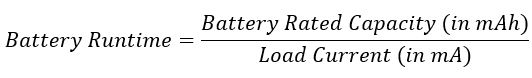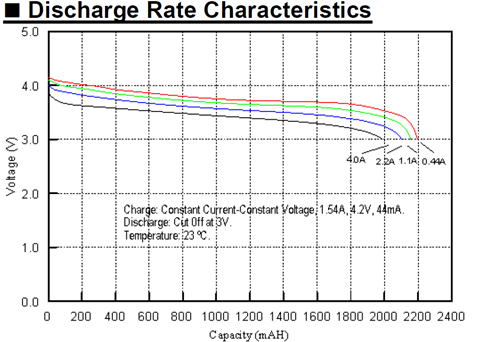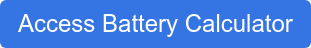Itamar Frankenthal January 18, 2022 10 min read

# How to Calculate 18650 Lithium-Ion Battery Pack Capacity and Runtime

## How to Calculate a Lithium-Ion Battery Pack's Capacity and Runtime

Capacity Varies With Load Current - Batteries have a nominal capacity, but their real capacity depends on the current being drawn from them.

Capacity is a function of the type of battery you are using, the load current, temperature and age of the cell. The capacity of lithium-ion batteries can be reduced by as much as 25% at high current (C rating) and operating temperature as compared to their published capacity. Manufacturers typically publish the the capacity when the load is C/5 or one fifth of the rated capacity. When you are trying to determine the capacity at your use conditions, a bit more engineering is required.

Batteries have internal impedance which reduces capacity and runtime at high load currents. Basic battery calculators don't account for the reduction in capacity. The best solution is to generate empirical cycling data at the desired current or use an advanced battery calculator that accounts for the cell's unique impedance profile.

#### Cell Capacity 101:

What is a Cell's Rated Capacity? A Lithium Ion battery’s published rated capacity is the capacity of the cell when the load current is one fifth of the rated capacity (the C Rate). When the current varies from C/5, the capacity will change due to chemical reaction rates including a chemical effect called concentration polarization.

What Is the Capacity of a Cell When the Load Current Increases ? If the load current is greater than one fifth of rated capacity, the capacity and runtime decreases. The cell's capacity will be less than the rated capacity.

What Is the Capacity of a Cell When the Load Current Decreases? If the load current is lower than the rated capacity, the capacity and runtime increases.The cell's capacity will be grater than the rated capacity.

#### Method 1 - Standard Battery Calculators

Standard online battery calculators us the formulaBenefits of Standard Battery Calculators - The calculator is very helpful because it provides a first order approximation for the runtime. You can experiment with our standard calculator here.

Limitations of Standard Battery Calculators - This formula is only an approximation because it uses the same rated capacity regardless of the load current. The actual capacity of a cell varies with the load current.

#### Method 2 - Use a Cell Data Sheet Discharge Curve

Use a cell data sheet (like this one for the MoliCell ICP103450DA 2200 mAh Cell) for the specif cell you are assessing. Use the discharge curve to approximate the capacity at the desired load current then use the formula Runtime = Capacity at Load Current/Load Current

Advantages of Discharge Curves - When available, discharge curves provide a more precise calculation for runtime as they are based on empirical data.

Limitations of Discharge Curves - Even when vendors provide data sheets, they may not be the most helpful. For example, the LG INR 21700 M50LT and Samsung NR21700-50E are very popular Lithium-Ion 21700 cells with ~4900 mAh capacity. Their data sheets only contain the cell's capacity at the standard discharge rate of one firth of the rated capacity (know as C/5). Further, Discharge curves only show a few different load profiles. For load profiles not displayed, the capacity can only be approximated. They only show the capacity of a fresh battery and don't show how the capacity desegregates over time.

#### Method 3 - Use an Advanced Lithium-Ion Battery Pack Calculator

Advanced battery pack runtime calculators account for internal impedance by utilizing empirical cell cycling data to provide a more accurate runtime calculation. Each cell has a unique chemical profile which gets captured through cell cycling and uploaded to a database. The advance calculator uses the cell's impedance profile to calculate the capacity at the load current. You can try our Advanced 18650 Lithium-Ion Battery Pack Calculator.

Advantage of Advance Battery Pack Calculators - Advanced battery pack calculators are a great way to get a quick answer. You can experiment with "what if" scenarios and compare different cells at the same time.

Limitations of Advance Battery Pack Calculators - Advanced Battery Pack Calculators require a large database of cell cycling data. Often times, the cell you are considering may not be part of the dataset. The result is based on a mathematical model and therefore has some variance from the true capacity. Advanced battery pack calculators are therefore best used for engineers looking to understand how different batteries behave under varying load conditions.

#### Method 4 - Use Empirical Cell Cycling Data

The most precise way to determine a cell's runtime is to cycle multiple cells on a battery analyzer under the same conditions the cell will be used. Analyzers can be run with varying currents, constant current loads, constant power loads, and pulse currents. The resulting data will provide both the voltage curve across time and the variance from cells within a lot.

Advantages of Empirical Cell Cycling Data - Cycling data will provide the most accurate results. Cycling a battery hundreds of time can also provide data on how capacity desegregates with use. Using a thermal chamber, cycling studies can be performed at varying temperatures.

Limitations of Empirical Cell Cycling Data - The greatest limitation is time and cost. Cell cycling data, especially under low currents can take weeks or months to generate.

### Example: MoliCell's ICP103450DA 2200 mAh Cell

The MoliCell ICP103450DA is a very popular prismatic cell. It’s rated capacity is 2200 mAh. As we can see from the below discharge rate characteristics, this capacity is only at 0.44A, which is one fifth the rated capacity. At a load of 0.44A, the cell will have a capacity of 2200 mAh and a runtime of 5 hours (2200 mAh divided by 440 mA)Source: MoliCell

What if we increase the load current to 4A? What will happen to the runtime?

• Method 1: Using a Standard Battery Calculator - If we use the standard battery calculator formula, we would use the rated capacity of 2200 mAh, calculate the runtime as 2200 mAh divided by 4000 mA and conclude the runtime is 0.55 hours or 33 minutes. This is only an approximation.
• Method 2: Using Use a Cell Data Sheet Discharge Curve

As we can see from the chart and the datasheet, under a 4A load, the battery capacity decreases to ~1980 mAh. The runtime will therefore be 1980 mAh divided by 4000 mAh which is ~0.4950 hours or ~29.7 minutes. The capacity decreased because the high current increases the internal impedance.

• Method 3: Using an Advance Battery Pack Calculator

Advance battery pack calculators use empirical data. Under lab conditions the the capacity may be closer to 1982 mAh. The runtime will therefore be 1982 mAh divided by 4000 mAh which is ~0.4955 hours or ~29.7 minutes. The capacity decreased because the high current increases the internal impedance.

Method 2 produces a similar result to method 3 because both use empirical data

• Method 4: Using Empirical Cell Cycling Data

Under lab conditions the the capacity may be closer to 1982 mAh. The runtime will therefore be 1982 mAh divided by 4000 mAh which is ~0.4955 hours or ~29.7 minutes. The capacity decreased because the high current increases the internal impedance.

Method 2, 3 and 4 will produce similar results because they use empirical data

### Conclusion

A basic battery calculator is a great way to get a first pass approximate calculation for battery runtime. As the load current varies from one fifth of the rated capacity, the calculation becomes less accurate. The best way to calculate runtime is using empirical data that is unique to the cell, because each cell has a different non-linear impedance profile. Empirical data can be obtained using a data sheet, Advanced 18650 Battery Pack Calculators or cell cycling.